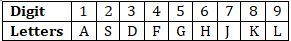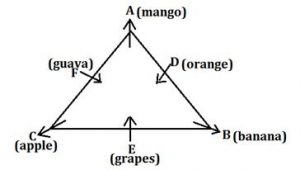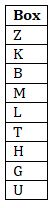Latest Banking jobs   »   Reasoning Ability Quiz For IBPS Clerk...

# Reasoning Ability Quiz For IBPS Clerk Prelims 2021- 19th August

Directions (1-5): Study the information carefully and answer the questions given below:
Six persons are sitting around a triangular table such that three of them sit at the corners and the rest on the middle of the side. The one who sits at the corner face away from the center and the one who sits at the middle of the side face towards the center. They also like different fruits. A sits second to the left of the one who likes banana. Only two persons sit between C and D. F sits second to the left of the one who likes grapes. C sits second to the right of B. Neither F nor C likes banana. The one who likes mango sits second to the right of the one who likes apple. D sits middle of the side and likes orange. Neither A nor E likes guava.

Q1. Who among the following likes guava?
(a) B
(b) C
(c) D
(d) E
(e) F

Q2. Who among the following sits immediate right of the one who likes banana?
(a) A
(b) C
(c) The one who likes grapes
(d) The one who likes orange
(e) F

Q3. How many persons sit between A and C, when counted right of A?
(a) None
(b) Two
(c) Three
(d) One
(e) More than three

Q4. What is the position of D with respect to F?
(a) Immediate left
(b) Third to the right
(c) Immediate right
(d) Third to the left
(e) Second to the left

Q5. Four of the following five from a group, which among the following does not belong to this group?
(a) F-Mango
(b) A-Grapes
(c) E-Apple
(d) B-Orange
(e) D-Banana

Directions (6-10): Study the information carefully and answer the questions given below.
Nine boxes are placed one above others in a stack. Four boxes are placed between box H and K. Box K is placed above box H. One box is placed between Box H and L. Three boxes are placed between box M and G. Two boxes are placed between Box U and T. Box Z is placed above box B. Box M is not placed just above or just below box K. Box M is placed above box H. Box K is not placed at topmost of stack. Box B placed just above box M. Box T is not placed at the bottom of the stack.

Q6. Which of the following box is placed just above box L?
(a) B
(b) M
(c) T
(d) H
(e) None of these

Q7. How many boxes are placed in between B and G?
(a) Four
(b) One
(c) Two
(d) Three
(e) None

Q8. Which of the following box is placed above box Z?
(a) One
(b) Two
(c) Four
(d) Three
(e) No one

Q9. Four of the following five are alike in a certain way based form a group, find the one that does not belong to that group?
(a) K-M
(b) B-L
(c) T-U
(d) Z-B
(e) G-T

Q10. If box B and box T interchanged their position then which of the following box is placed just below box B?
(a) M
(b) H
(c) L
(d) G
(e) None of these

Directions (11-15): In each of the questions given below, a group of digits is given followed by some combinations of letters. You have to find out which of given combinations correctly represents the group of digits based on the letters codes and the conditions given below. If none of the given combinations represents the group of digits correctly, give (e) i.e. ‘None of these’ as the answer.Condition for coding the group digits:
(i) If the first digit is odd and last digit is even, the codes for the first and the last digits are to be interchanged.
(ii) If the first as well as the last digit is even, both are to be coded by the code for the last digit.
(iii)If the first as well as the last digit is odd, both are to be coded by the code for the first digit.
Q11. 2658478
(a) SHGKFJK
(b) KHGKFJK
(c) KHGKJFK
(d) KHGKFJG
(e) None of these

Q12. 3654878
(a) KHGFKJK
(b) DHGFKJD
(c) KHFGKJD
(d) KHGFKLD
(e) None of these

Q13. 6792479
(a) HJLSFJH
(b) HJSLFJL
(c) HJLSJFL
(d) HJLSFJL
(e) None of these

Q14. 1234567
(a) ASDFGHJ
(b) ASDFGHA
(c) JSDFGHJ
(d) ASDGFHA
(e) None of these

Q15. 9876542
(a) SKJJHGL
(b) LKJHGFS
(c) SKJHGFL
(d) LJKHGFS
(e) None of these

Solutions

Solutions (1-5):
Sol.S1. Ans.(e)
S2. Ans.(c)
S3. Ans.(c)
S4. Ans.(e)
S5. Ans.(b)

Solutions (6-10):
Sol.S6.Ans.(b)
S7.Ans.(a)
S8.Ans.(e)
S9.Ans.(c)
S10.Ans.(b)

S11. Ans.(b)
Sol. Condition (ii) applies

S12. Ans.(e)
Sol. Conditions(i) applies.

S13. Ans.(d)
Sol. No Condition are applied.

S14. Ans.(b)
Sol. Condition (iii) applies.

S15. Ans.(c)
Sol. Conditions(i) applies.#### Congratulations!Union Budget 2023-24: Free PDF